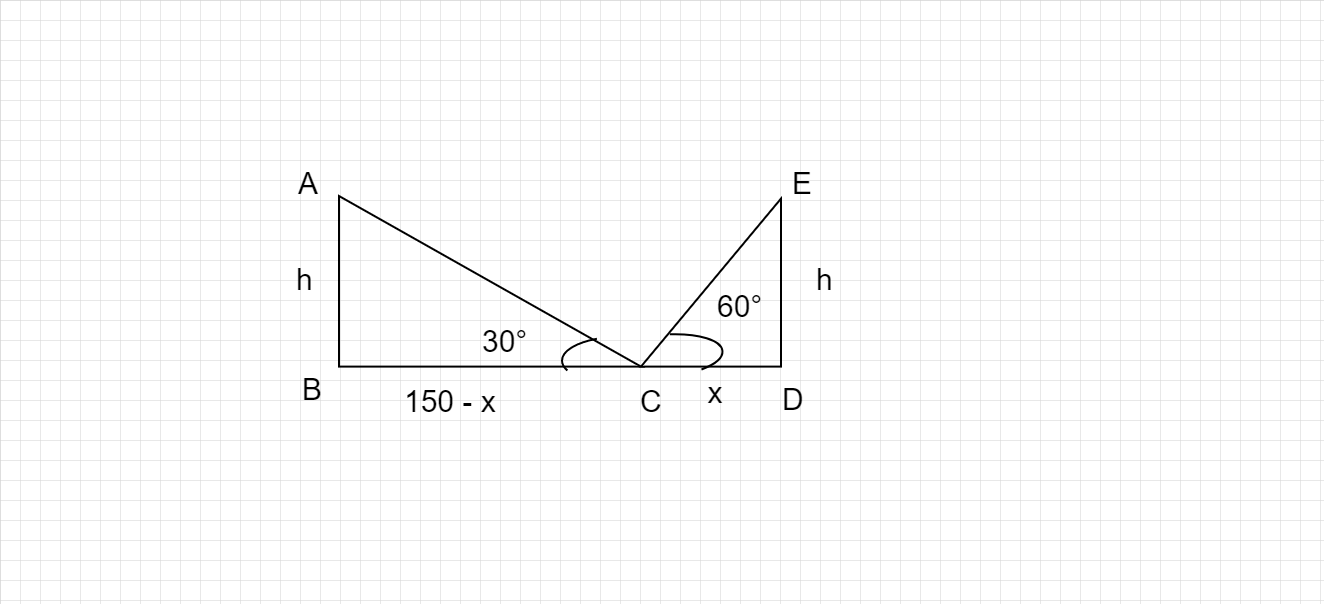# Two pillars of equal heights stand on either side of a roadway, which is 150 m wide. At a point in the roadway between the pillars the elevations of the tops of the pillars are 60° and 30°. Find the height of the pillars and the position of the point.

Given :

Width of Road = 150 m

Angle of elevations of pillars = 60° and 30°

To Find :

Position of point from the pillars

Height of Pillars

Solution :Lets take the Height tower  = h

Position of point is at C.

BD  =  150 m

CD  =  x   ;  BC  =  150 - x

In ABC

$$\displaystyle tan\ 30° \ =\ \frac{h}{150-x} \ \ \ \ \ \ \ \ \ \ \ \ \ \ \ \ \ \ \ \ \ \ \ \ \ \ \ \ \ \ \ \ \ \ \ \ \ \ \ \ \ \ \ \ \ \ \ \ \ \ \ \ \ \ tan\ \theta \ =\ \frac{opposite}{hypotenusse}$$

$$\displaystyle \frac{1}{\sqrt{3}} \ =\ \frac{h}{150-x} \ \ \ \ \ \ \ \ \ \ \ \ \ \ \ \ \ \ \ \ \ \ \ \ \ \ \ \ \ \ \ \ \ \ \ \ \ \ \ \ \ \ \ \ \ \ \ \ \ \ \ \ \ \ tan\ 30° \ =\ \frac{1}{\sqrt{3}}$$

$150 - x \ \ = \ h √3$............................................(i)

In EDC

$$\displaystyle \begin{array}{{>{\displaystyle}l}} tan\ 60° \ =\ \frac{h}{x} \ \ \\ \\ \sqrt{3\ } \ =\ \frac{h}{x} \ \ \ \ \ \ \ \ \ \ \ \ \ \ \ \ \ \ \ \ \ \ \ \ \ \ \ \ \ \ \ \ \ \ \ \ \ \ \ \ \ \ \ \ \ \ \ \ \ \ \ \ \ \ \ \ \ \ \ \ \ \ \ \ \ \ \ \ \ \ \ \ \ \ \ \ \ \ tan\ 60° \ =\ \sqrt{3} \ \ \end{array}$$

$x √3 = h$

$h = x √3............................................................. (ii)$

Substitute (ii)  in  (i)

$150 - x \ = \ (x√3) √3$

$150 - x \ = \ 3 x \ \ \ \$

$150 = 3 x + x$

$150 = 4 x$

$4 x \ = \ 150 \$

$$\displaystyle \begin{array}{{>{\displaystyle}l}} x\ \ =\ \ \frac{150}{4} \ \\ \\ x\ \ =\ \ 37.5\ \end{array}$$

$150 - x \ = \ 150 - 37.5 \ = \ 112.5$

Substitute x = 37.5 in (ii)

h  =  37.5√3

so,

Position of point from the pillars  BC  =  112.5 m  ,  CD  =  37.5 m.

Height of the Pillars h = 37.5√3 m

Updated on: 10-Oct-2022

46 Views